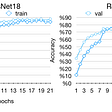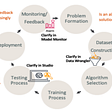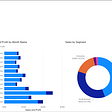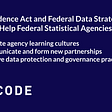# Introduction

In this articles series, I will focus on various visualizations and identify which visualization is best for showing certain information for a given datasets. I will describe every visualization in detail and give practical examples, such as comparing different stocks over time or comparing the ratings for different movies. Starting with comparison plots, which are great for comparing multiple variables over time, we will look at their types (such as line charts, bar charts, and radar charts).

I will then move onto relation plots, which are handy for showing relationships among variables. I will cover scatter plots for showing the relationship between two variables, bubble plots for three variables, correlograms for variable pairs, and finally, heat maps for visualizing multivariate data.

The article series will further explain composition plots (used to visualize variables that are part of a whole), as well as pie charts, stacked bar charts, stacked area charts, and Venn diagrams. To give you a deeper insight into the distribution of variables, we will discuss distribution plots, describing histograms, density plots, box plots, and violin plots.

Finally, i will talk about dot maps, connection maps, and choropleth maps, which can be categorized into geoplots. Geo plots are useful for visualizing geospatial data.

# Comparison Plots

Comparison plots include charts that are ideal for comparing multiple variables or variables over time. Line charts are great for visualizing variables over time. For comparison among items, bar charts (also called column charts) are the best way to go. For a certain time period (say, fewer than 10-time points), vertical bar charts can be used as well. Radar charts or spider plots are great for visualizing multiple variables for multiple groups.

# 1. Line Chart

Line charts are used to display quantitative values over a continuous time period and show information as a series. A line chart is ideal for a time series that is connected by straight-line segments.

The value being measured is placed on the y-axis, while the x-axis is the timescale.

• Line charts are great for comparing multiple variables and visualizing trends for both single as well as multiple variables, especially if your data set has many time periods (more than 10).
• For smaller time periods, vertical bar charts might be the better choice.

The following diagram shows a trend of real estate prices (per million US dollars) across two decades. Line charts are ideal for showing data trends:

The following figure is a multiple-variable line chart that compares the stock-closing prices for Google, Facebook, Apple, Amazon, and Microsoft. A line chart is great for comparing values and visualizing the trend of the stock. As we can see, Amazon shows the highest growth:

# 2. Bar Chart

In a bar chart, the bar length encodes the value. There are two variants of bar charts: vertical bar charts and horizontal bar charts.

While they are both used to compare numerical values across categories, vertical bar charts are sometimes used to show a single variable over time.

• Don’t confuse vertical bar charts with histograms. Bar charts compare different variables or categories, while histograms show the distribution for a single variable. Histograms will be discussed later in this chapter.
• Another common mistake is to use bar charts to show central tendencies among groups or categories. Use box plots or violin plots to show statistical measures or distributions in these cases.

The following diagram shows a vertical bar chart. Each bar shows the marks out of 100 that 5 students obtained in a test:

The following diagram shows a horizontal bar chart. Each bar shows the marks out of 100 that 5 students obtained in a test:

The following diagram compares movie ratings, giving two different scores. The Tomato meter is the percentage of approved critics who have given a positive review for the movie. The Audience Score is the percentage of users who have given a score of 3.5 or higher out of 5. As we can see, The Martian is the only movie with both a high Tomato meter and Audience Score. The Hobbit: An Unexpected Journey has a relatively high Audience Score compared to the Tomato meter score, which might be due to a huge fan base

Design Practices

• The axis corresponding to the numerical variable should start at zero. Starting with another value might be misleading, as it makes a small value difference look like a big one.
• Use horizontal labels-that is, as long as the number of bars is small, and the chart doesn’t look too cluttered.
• The labels can be rotated to different angles if there isn’t enough space to present them horizontally. You can see this on the labels of the x-axis of the preceding diagram.

Radar charts (also known as spider or web charts) visualize multiple variables with each variable plotted on its own axis, resulting in a polygon. All axes are arranged radially, starting at the center with equal distances between one another, and have the same scale.

• Radar charts are great for comparing multiple quantitative variables for a single group or multiple groups.
• They are also useful for showing which variables score high or low within a dataset, making them ideal for visualizing performance.

The following diagram shows a radar chart for a single variable. This chart displays data about a student scoring marks in different subjects:

The following diagram shows a radar chart for two variables/groups. Here, the chart explains the marks that were scored by two students in different subjects:

The following diagram shows a radar chart for multiple variables/groups. Each chart displays data about a student’s performance in different subjects:

Design Practices

• Try to display 10 factors or fewer on a single radar chart to make it easier to read.
• Use faceting (displaying each variable in a separate plot) for multiple variables/groups, as shown in the preceding diagram, in order to maintain clarity.

In this blog, we learned which plots are suitable for comparing items. Line charts are great for comparing something over time, whereas bar charts are for comparing different items. Last but not least, radar charts are best suited for visualizing multiple variables for multiple groups. In the following activity, you can check whether you understood which plot is best for which scenario. Check the next article in this visualization series for more graphs.

For more awesome content and regular posts you can connect with me on Instagram😍

--

--

--

## More from Abhijeet Srivastav

CEO Techneophyte | Python Developer | ML Engineer | Data Scientist | Flutter Developer | Penetration Tester | Software Engineer at Infosys

Love podcasts or audiobooks? Learn on the go with our new app.

## [Week5–6 — Where is this, in Ankara?]## Exploring French MPs Financial Assets with Python and OpenRefine## Sage Maker & Sage Maker Clarify## The rise of data apps & how to build apps in a blazing-fast way## Content of tables## Create your first report in Power BI## Future of the Metrics Layer with Drew Banin (dbt) and Nick Handel (Transform)## Implementing federal data policies by statistical agencies## Abhijeet Srivastav

CEO Techneophyte | Python Developer | ML Engineer | Data Scientist | Flutter Developer | Penetration Tester | Software Engineer at Infosys

## Geometric Mean using Pandas in Python## Matplotlib in Python## How to Create Plots With Pandas and Matplotlib — Andres Berejnoi